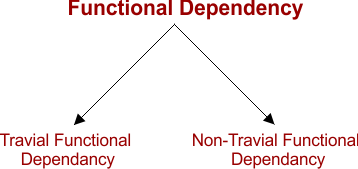Select Page

# Functional Dependency (FD)

The functional dependency (FD) is a relationship that exists between two attributes, Where one attribute can directly or indirectly derived from the other attribute.

Syntax:

X   →   Y

The left side of FD (attribute X) is known as a determinant, the right side of the FD (attribute Y) is known as a dependent. So “Y” can determined from “X”.

## Types of FD

There are two types of functional dependency

• Trivial FD
• Non Trivial FD#### Let explain with example:

Suppose we have an Student table with attributes: Student_Id, Student_Name, Student _class.

Here Student_Id attribute can uniquely identify the Student_Name and Student_address attribute of Student table.

### 1. Trivial FD

• A → B has trivial functional dependency if B is a subset of A or B.
• it is the case where the derived attribute is derived directly
• The following dependencies are also trivial like: A → A, B → B

Example:

• {Student_id, Student_Name}   → Student_Id  // it is a trivial functional dependency as Student_Id is a subset of { Student_Id, Student_Name}.
• Also, Student_Id → Student_Id and Student_address →   Student_address are trivial dependncies

#### Keep In Mind

• Intersection of left hand side of FD and right hand side of FD will never be a null
• L.H.S ∩ R.H.S ≠ Ø
• Travail FD are valid in each case and never be a problematic in transactions

### 2. Non-Trivial FD

• A → B has a non-trivial functional dependency if B is not a subset of A.
• it is the case where the derived attribute are not derived directly

Example:

As in the following example, Student_address is derive from student_ID but not directly

• Student_ID   →    Student_Name

#### Keep In Mind

• When ‘A’ intersection ‘B’ is NULL, then A → B is called as complete non-trivial.
• Intersection of both left and right side will always be null (A ∩ B = Ø)
• Non-travial are not valid in each case

Help Other’s By Sharing…